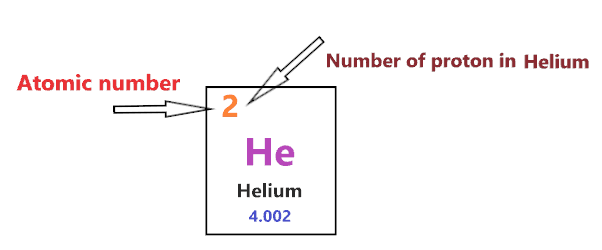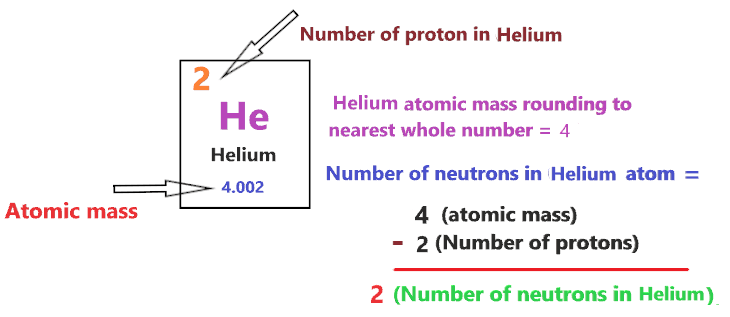# Bohr model of Helium atom - How to draw Helium(He) Bohr-Rutherford diagram?

Home  > Chemistry Article > Helium Bohr model

The Bohr Model of Helium(He) has a nucleus that contains 2 neutrons and 2 protons. This nucleus is surrounded by an electron shell named K-shell. The outermost shell in the Bohr diagram of Helium contains 2 electrons that also called valence electrons.

 Name Helium Bohr Model Number of neutrons 2 Number of protons 2 Number of electrons 2 Total electron shells 1 Electron in the First shell(K) 2 Total valence electrons in Helium 2
Page Contents

## How to draw Bohr Model of Helium(He)?

Bohr model describes the visual representation of orbiting electrons around the small nucleus. It used different electron shells such as K, L, M, N…so on. These shells hold a specific number of electrons, the electron shell which is closest to the nucleus has less energy and the electron shell which is farthest from the nucleus has more energy.

Bohr diagram is very interesting and easy to draw. Here, we will draw the Bohr diagram of the Helium atom with some simple steps.

## Steps to draw the Bohr Model of Helium atom

1. Find the number of protons, electrons, and neutrons in the Helium atom

Protons are the positively charged particles and neutrons are the uncharged particles, both these are constituents of the atom nuclei. Electrons are the negatively charged particles that orbit the nucleus of an atom

To find the number of protons an atom contains, just look at their atomic number.

If the atomic number of atom is 19, then proton will also be 19, if atomic number is 20, then proton will also be 20.

So, the atomic number for Helium is 2, hence, the number of protons in the Helium atom is also 2.Now, to determine the number of neutrons in an atom, use this formula.

⇒ Number of neutrons in atom = Atomic mass of the atom(rounded to the nearest whole number) – Number of proton in an atom

For example, An atom have 17.995 atomic mass and 9 protons.

Then, to find the number of neutron, round the atomic mass to the near whole number, so, atomic mass 17.995 round to 18.

= (18 – 9 protons) = 9 number of neutrons in the atom

Now, To get the number of neutrons in a Helium atom, look at its atomic mass which is 4.002 rounded to 4, and the number of protons in Helium is 2.

∴ Hence, the number of neutrons in Helium atom = (4 – 2) = 2It should be noted that “The number of electrons in a neutral atom is equal to the number of protons”.

So, the Helium atom is neutral, hence, its number of electrons will be equal to the number of protons which is two as we already discussed.

⇒ The number of electrons in Helium atom = 2

⇒ The number of protons in Helium atom = 2

⇒ The number of neutrons in Helium atom = 2

2. Draw the nucleus of an atom

A nucleus is a dense and small region that contains the number of protons and neutrons of an atom.

In this step, we have to draw a small circle that consists of a number of protons and the number of neutrons of a Helium atom.3. Draw the First electron shell

“An electron shell may be thought of as an orbit followed by electrons around an atom’s nucleus.”

The first electron shell is also called the K-shell, this is the closest shell to the nucleus of an atom and can hold a maximum of two electrons.

As we know, the Helium atom has a total of 2 electrons only. So, put these two electrons in the first shell, next to each other.### Bohr model of Helium atom

That’s all, this is our Bohr model of the Helium atom that contains 2 protons and 2 neutrons in the nucleus region, and 2 electrons in the first shell that orbited around the nucleus.

## Find Valence electron of Helium through its Bohr diagram

Valence electrons are found in the outermost shell of an atom and they can take participate in the formation of a chemical bond. These electrons have more energy compare to the inner shell electrons.

From the Bohr diagram of an atom, we can easily find the number of valence electrons in an atom by looking at its outermost shell.

So, we have to find a valence electron in the Helium atom, for this, look at its Bohr diagram.

Bohr’s diagram of Helium has only one electron shell (K-shell ).

Hence, the electrons found in the K-shell of the Helium atom are its valence electrons because it is the outermost shell that also called the valence shell.

The K-shell or outer shell of the Helium Bohr model contains 2 electrons, therefore, the number of valence electrons in the Helium atom is 2.## Electron dot diagram of Helium atom

Electron dot diagram also called lewis structure which represents the valence electrons of atoms.

As, from the Bohr diagram of Helium, we got to know, it has 2 valence electrons.

So, just represent these 2 valence electrons around the Helium atom as a dot.## The electron configuration of Helium

Electron configuration is the distribution of electrons of an atom or molecule in atomic or molecule orbitals”

Helium has an atomic number of 2 and it contains a total number of 2 electrons. From the Bohr model of Helium, we know, it has 2 electrons in the K-shell.

So, based on the shell, the electronic configuration of the Helium atom is .

Or the electronic configuration of Helium is 1s2 since it contains a total of 2 electrons and these two electrons will go in 1s orbital.

S orbital can hold maximum of 2 electrons.

## FAQ

### How many electron shells a Helium Bohr model contains?

Electron shell also called energy level, you can find the number of electron shells for an element by knowing its period number in the periodic table.

The elements or atoms in the first period of the periodic table have one energy level or one electron shell, same as, the elements in the second period have two energy levels or two-electron shells and so on…

So, the Helium atom belongs to the 1st Period in the periodic table, hence, the number of electron shells for the Bohr model of Helium will be only 1 (K-shell).

### What is the outer shell of the Bohr diagram of the Helium atom?

The outermost shell also called the valence shell, which contains valence electrons of the atom.

According to the Bohr diagram of Helium, the outer shell is K-shell which contains 2 valence electrons.

## Properties of Helium

1. It is non-toxic, colorless, odorless, and tasteless gas.
2. It belongs to the noble gas group in the periodic table.
3. It has a boiling point of −268.928 °C and a melting point of −272.20 °C.
4. It has a zero oxidation state.
5. It is the second lightest and second most abundant element in the universe.

## Summary

• The Bohr model of Helium is drawn with only one electron shell and it contains 2 electrons.
• Helium is neutral and its atomic number is 2, hence, the number of protons and electrons available for its Bohr diagram is also 2.
• The number of neutrons for the Bohr diagram of Helium can be found by subtracting the number of protons from the atomic mass(rounded to the nearest whole).
• The electron configuration of Helium in terms of the shell is , or in normal form, it is 1s2.
##### Subscribe to Blog via Email

Join 2 other subscribers

Share it...